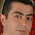## Sunday, August 15, 2010

### Problem 504: Equilateral Triangle, Angles, 45, 60 Degrees

Geometry Problem
Click the figure below to see the complete problem 504 about Equilateral Triangle, Angles, 45, 60 Degrees.Complete Problem 504

Level: High School, SAT Prep, College geometry

1.in triangle ABD:<A=45,<B=105,<D=30
with the sine law
BDsin30=ABsin45;BD=ABsqr(2)
in triangle BDC
with the cosine law
CD²=BC²+BD²-2BC.BD.cos45=AB²
CD=AC
triangle ACD is isoscele
x=15
.-.

2.let t=AB, it is known that BD=t\sqrt{2} (because tr ABD is 45-30-105).
Consecuently CD=t (by tracing CX, altitude of tr BCD and using Pithagorean Theorem).
So tr ACD is isosceles and finally <CDA= < CAD=15.

by mathreyes

3.draw DG perpendicular to AD, G on extensin of AC
ABDG cyclic AC = CB = R => C midpoint => AC = CD

4.arc BG = 2∙A = 120° => arc AB = 60° => C midpoint

5.C origin, A,B and D circular
IBCI=ICDI=r
so trianle BCD isosceles and x=15

6.Draw perpendiculars BP,CQ to AD,BD respectively. Then Tr ABP congruent to Tr BQC SAA. So BP =QC=BQ=QD since < BDP=30. So Tr BQC= Tr DQC. Hence <QDC=45. x=15

Sumith Peiris
Moratuwa
Sri Lanka

7.Draw perpendiculars BP and CQ to AD and BD respectively. Then these 2 peroenduculars are easily seen to be equal from congruent Tr.s. Also BP=CQ=BQ=1/2 BD (90 60 30 Tr) = QD, so Tr BQC =Tr BQD. Hence<CDQ = 45,'X=15

8.Problem 504
Is <ABD=60+45=105 ,so <BDA=30=60/2=(<BCA)/2. Then the point C is the circumcenter of triangle ABD.So AC=CD.Therefore <ADC=<DAC=60-45=15.
APOSTOLIS MANOLOUDIS 4 HIGH SHCOOL OF KORYDALLOS PIRAEUS GREECE

9.Solution2

Let the bisector of < ACB meet AD at E.
Then <BDE <BCE = 30, hence BECD is concyclic.
So x = <CBE = < CAE =15

Sumith Peiris
Moratuwa
Sri Lanka

10.Solution 3

Let X be the foot of the perpendicular from B to AD

If BD = 2p then since <BDX = 30, BX = AX = p and so AB =BC = sqrt2.a

So in Triangle BCD, <B = 45, BC = sqrt2.p and BD = 2p, hence the same is easily seen to be right angled at C.

Hence BDCX is concyclic and x = <XBC = 15

Sumith Peiris
Moratuwa
Sri Lanka